# SAT Math Multiple Choice Question 34: Answer and Explanation

### Test Information

Question: 34

4.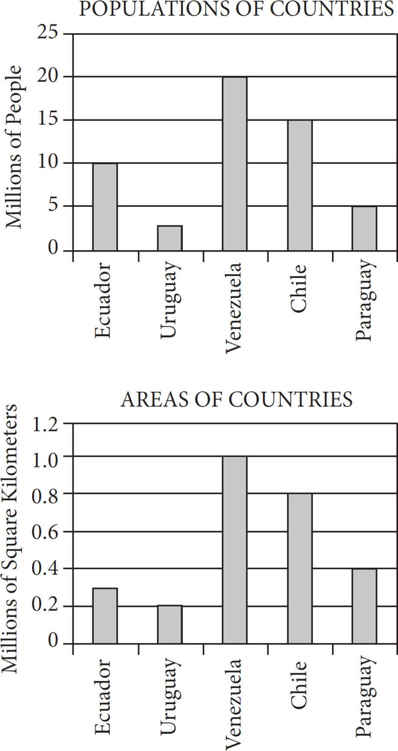The populations and areas of five countries are shown in the graphs above. If population density is defined as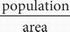, which of the five countries has the highest population density?

• B. Uruguay
• C. Venezuela
• D. Chile

Explanation:

A The top graph is of the countries' populations, and the bottom graph is of the countries' areas. Find the population density,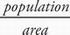, for each country by taking its number from the top graph and dividing that by its number from the bottom graph:

Ecuador =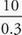, which equals 33.33

Uruguay =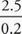, which equals 12.5

Venezuela =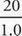, which equals 20

Chile =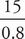, which equals 18.75

The highest value among the countries is that of Ecuador, which is (A).